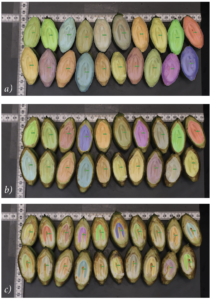Figure 1 shows the measurement method of the areas of shuck (a), shell (b) and embryo (c). To define the area of interest of each element (embryo, shell and shuck), an
algorithm was developed based on the Mask R-CNN, a convolutional neural network that works in two steps. The first step is the object detection, namely Faster R-CNN,
where the element is identified and a region of interest where it is inserted is defined. The
second step is the actual Mask R-CNN, where the bounds and the area of the element is
defined. The area given in number of pixels is later transformed to millimeters squared
using a metric tape contained on all pictures, where the size of each pixel is measured by
counting the number of pixels there is in a 10 millimeters distance.